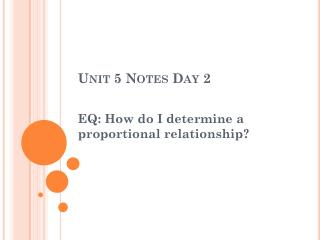DownloadDownload PresentationUnit 5 Notes Day 2

Unit 5 Notes Day 2

Télécharger la présentationUnit 5 Notes Day 2

- - - - - - - - - - - - - - - - - - - - - - - - - - - E N D - - - - - - - - - - - - - - - - - - - - - - - - - - -
Presentation Transcript

1. Unit 5 Notes Day 2 EQ: How do I determine a proportional relationship?

2. Proportional vs. Non-Proportional • If two quantities are proportional, then they have a constant ratio. • If the ratio is not constant, the two quantities are said to be non-proportional.

3. Vocabulary • Proportional: For a relationship to be constant there has to be a constant ratio. The graph of a proportional relationship will cross through the origin (0,0) • Nonproportional: No constant ratio. The graph will not cross the origin.

4. Is it Proportional? • Uptown pizzeria sells medium pizzas for \$7 each but charges a \$3 delivery fee per order. Is the cost of an order proportional to the number of pizzas ordered? • Find the cost for 1,2,3,and 4 pizzas and make a table to display numbers and cost.

5. At the beginning of the school year, Isabel had \$120 in the bank. Each week, she deposits another \$20. Is her account balance proportional to the number of weeks since she started school?

6. Proportional Relationships • Will always go through the origin on a graph. (0,0) • Graph will always be a straight line. • Always write the constant ratio in the form of • Reduce or divide to find the constant ratio for each.

7. Proportional or Non-proportional? • In order to tell from a table if there is a proportional relationship or not, you can check to see if the ratio is the same. • The ratio is also known as the scale factor.

8. EX 1. and 2.) Tell if the following tables represent a proportional relationships. Proportional? ______ Proportional? Ratio Ordered Pair Ratio _________ Equation____________ Ordered Pair _________

9. In order to tell if a graph is proportional the line must go through the origin. EX 3. and 4.) Tell if the following graphs represent a proportional relationships. Proportional ? _________ Proportional ? _________ Line does not go thru the origin Why? Why? Line goes thru the origin

10. In order to tell if a set of ordered pairs is proportional, look at the ratio of y to x. EX 5.) Tell if the following set of ordered pairs represents a proportional relationship. Proportional? ______ Equation ___________ Ordered Pair _________ Ratio _________

11. EX 6. and 7.) Determine if the following equations show a proportional relationship. Put a zero in for x; if y is zero then it is a proportional relationship because it goes through the origin. y = 10x y = 3x – 1

12. 8. At HPMS, there are 26 students per teacher in advisory. Is the total number of students proportional to the number of teachers?

13. 9. The City Pool costs \$8 per day to visit during the summer. There is also a \$25 yearly registration fee. Is the total cost proportional to the total number of days visited?

14. Equations: • We will also make equations to describe each situation. • If the situation is proportional, you will use your constant ratio in your equation. • Make sure you define your variables!!!

15. Example 1 Identify Proportional Relationships • Sal’s Pizzeria sells large pizzas for \$11 but charges a \$2 delivery fee per order. • Write an equation to fit this situation:

16. Example 1 Sal’s Pizzeria sells large pizzas for \$11 but charges a \$2 delivery fee per order.

17. Write an equation that fits this situation: Hint: Use your constant ratio!! Ex. 2) A lemonade recipe calls for 1.5 cups of lemon juice and 6 cups of cold water.

18. Example 2 A lemonade recipe calls for 1.5 cups of lemon juice and 6 cups of cold water.